# How to Calculate Wire Diameter & Cross-Sectional Area

In this blog, we will review the concept of resistance, resistivity and steps to calculate the minimum cross-sectional area and diameter of any desired conductor.

## What is Resistance?

The property of a device or a circuit that opposes the movement of current through it. Resistance is measured in Ohms (Ω). The resistance of any material with a uniform cross-sectional area is determined by the following four factors:

1. The kind of material
2. The length
3. The cross-sectional area
4. The temperature

## What is Resistivity?

Resistivity is the measure of how much a given size of specific material resists the current flow. While materials resist electrical current flow, some are better at conducting it than others. Resistivity is used to compare the inherent resistance characteristics of different materials. Materials that easily conduct current are referred to as conductors. Conductors have a low resistivity. While the materials that do not easily conduct current are referred to as insulators. Insulators have a high resistivity. The resistivity of a material plays an important role in choosing the materials used for electric wire.

Now that we understand the concept of resistance and resistivity clearly, let’s review the general relationship of basic conductor resistance, which suggests that the resistance of a given conductor is equal to the material resistivity times the ratio of its length to its cross-sectional area. This can help us to calculate the minimum cross-sectional area and diameter of any desired conductor.

Let’s work out an example to understand the steps to calculate the minimum cross-sectional area and diameter of any desired conductor.

Example: What is the minimum cross-sectional area and diameter of conductor for a 750-meter-long copper wire having a maximum resistance of 0.2 ohms?

## Minimum Cross-sectional Area:

To solve this problem, we will use the general relationship for calculating conductor resistance using the formula below:

Resistance = Resistivity * (Length / Area )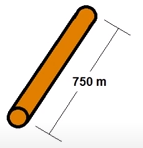R =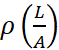R = Resistance of material, in Ohms

Ρ = Material Resistivity, in Ohms per meter

L = Conductor Length, in meters

A = Cross-sectional Area, in square meters

In order to use this general relationship to solve our example problem, we require the specific resistance or resistivity of copper.  Note that we acquire the resistivity of conductor materials from the table of conductor resistivity and we know now that copper has a resistivity of 1.72 x 10e-8 ohms per meter.

While solving Conductor Resistance, remember to express resistance in ohms, material resistivity in ohms per meter, conductor length in meters, and cross-sectional area in square meters for this relationship to be valid. We can then move on to calculate the cross-sectional area of the wire by substituting for the known quantities in the example.

A =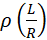Eq. (1)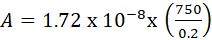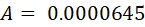## Diameter of the conductor:

Area of a circle can be represented using below formula. We will have to rearrange the formula to solve for diameter.

A =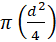4*A =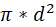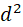=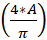d =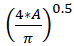We can now substitute our acquired cross-sectional area value from Eq. (1) into this relationship and calculate the diameter of the copper wire to arrive at a diameter of 0.009062 meters.

d =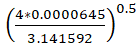d = (8.212395*10-5)0.5

d = 0.009062 m

Now that we have the solution values, we will convert them and express our final answer using the standard units.

A = 0.0000645 m2 = 64.5 mm2

d = 0.009062 m = 9.062 mm

Thereby, we conclude that our copper wire must have a minimum cross-sectional area of no less than 64.5 mm^2 and a diameter of no less than 9.062 mm.